# Regular group

(diff) ← Older revision | Latest revision (diff) | Newer revision → (diff)

There are several (different) notions of regularity in group theory. Most are not intrinsic to a group itself, but pertain to a group acting on something.

## Regular group of permutations.

Letbe a finite group acting on a set, i.e. a permutation group (group of permutations). The permutation groupis said to be regular if for all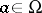,, the stabilizer subgroup at, is trivial.

In the older mathematical literature, and in physics, a slightly stronger notion is used:is transitive (i.e., for all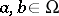there is a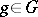such that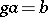) and, where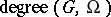is the number of elements ofandis, of course, the number of elements of. It is easy to see that a transitive regular permutation group satisfies this condition. Inversely, a transitive permutation group for whichis regular.

A permutation is regular if all cycles in its canonical cycle decomposition have the same length. Ifis a transitive regular permutation group, then all its elements, regarded as permutations on, are regular permutations.

An example of a transitive regular permutation group is the Klein-group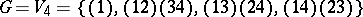of permutations of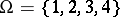.

The regular permutation representation of a groupdefined by left (respectively, right) translation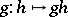(respectively,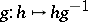) exhibitsas a regular permutation group on.

## Regular group of automorphisms.

Letact on a groupby means of automorphisms (i.e., there is given a homomorphism of groups,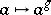,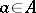).is said to act fixed-point-free if for allthere is asuch that, i.e. there is no other global fixed point except the obvious and necessary one. There is a conjecture that ifacts fixed-point-free onand, thenis solvable, [a7]; see also Fitting length for some detailed results in this direction.is said to be a regular group of automorphisms ofif for all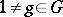only the identity element ofis left fixed by, i.e.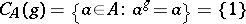for all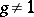. Some authors use the terminology "fixed-point-free" for the just this property.

## Regular-group.

A-group is said to be regular if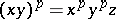, whereis an element of the commutator subgroup of the subgroup generated byand, i.e.is a product of iterated commutators ofand. See [a5].

How to Cite This Entry:
Regular group. Encyclopedia of Mathematics. URL: http://encyclopediaofmath.org/index.php?title=Regular_group&oldid=11804
This article was adapted from an original article by M. Hazewinkel (originator), which appeared in Encyclopedia of Mathematics - ISBN 1402006098. See original article